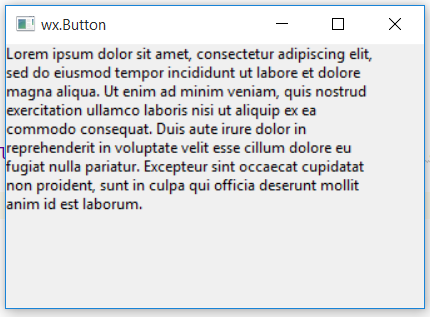# wxPython – Wrap() function in wx.StaticText()

• Last Updated : 18 Jun, 2020

In this article we are going to learn about Wrap() function associated with wx.StaticText class of wxPython. Wrap() functions wraps the controls label so that each of its lines becomes at most width pixels wide if possible (the lines are broken at words boundaries so it might not be the case if words are too long).

If width is negative, no wrapping is done. Note that this width is not necessarily the total width of the control, since a few pixels for the border (depending on the controls border style) may be added.

Attention geek! Strengthen your foundations with the Python Programming Foundation Course and learn the basics.

To begin with, your interview preparations Enhance your Data Structures concepts with the Python DS Course. And to begin with your Machine Learning Journey, join the Machine Learning - Basic Level Course

Syntax: wx.StaticText.Wrap(self, width)

Parameters:

ParameterInput TypeDescription
widthintwidth for wrapping.

Code Example:

 `import` `wx`` ` ` ` `class` `Example(wx.Frame):`` ` `    ``def` `__init__(``self``, ``*``args, ``*``*``kwargs):``        ``super``(Example, ``self``).__init__(``*``args, ``*``*``kwargs)``        ``self``.InitUI()`` ` `    ``def` `InitUI(``self``):``        ``self``.locale ``=` `wx.Locale(wx.LANGUAGE_ENGLISH)``        ``self``.pnl ``=` `wx.Panel(``self``)`` ` `        ``bmp ``=` `wx.Bitmap(``'right.png'``)``        ``# CREATE STATICTEXT AT POINT (20, 20)``        ``self``.st ``=` `wx.StaticText(``self``.pnl, ``id` `=` `1``, label ``=``"Lorem ipsum ... laborum."``, pos ``=``(``0``, ``0``),``                                 ``size ``=` `wx.DefaultSize, style ``=` `0``, name ``=``"statictext"``)`` ` `        ``# WRAP TEXT IN A PARTICULAR WIDTH``        ``self``.st.Wrap(``300``)`` ` `        ``self``.SetSize((``350``, ``250``))``        ``self``.SetTitle(``'wx.Button'``)``        ``self``.Centre()`` ` `def` `main():``    ``app ``=` `wx.App()``    ``ex ``=` `Example(``None``)``    ``ex.Show()``    ``app.MainLoop()`` ` ` ` `if` `__name__ ``=``=` `'__main__'``:``    ``main()`

Output Window:My Personal Notes arrow_drop_up